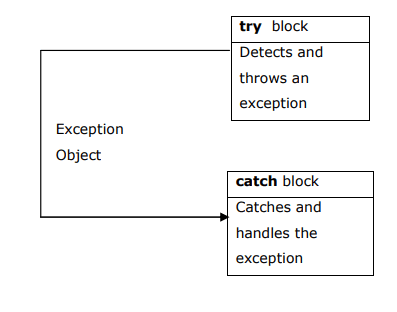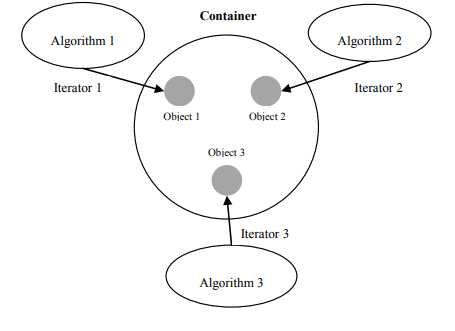# OOP Using C++ – C++ Programming Templates ,Exceptions and STL based Questions and Answers A+ A  A-

• 1. Explain Function and Class Templates with appropriate example.
• 2. Explain class template. Also write a C++ program for class template with multiple parameters.
• 3. What is exception handling? Explain how to handle an exception with appropriate example. OR Explain how to handle exceptions using try, catch and throw mechanism.
• 4. Explain multiple catch statements with appropriate example.
• 5. Explain Rethrowing an Exception with appropriate example.
• 6. Explain short introduction of STL
• 7. List out components of STL and explain in short

Question – 1. Explain Function and Class Templates with appropriate example.

• C++ templates are a powerful mechanism for code reuse, as they enable the programmer to write code that behaves the same for any data type.
• By template we can define generic classes and functions.
• It can be considered as a kind of macro. When an object of a specific type is defined for actual use, the template definition for that class is substituted with the required data type.

Function Template:

Suppose you write a function printData:

```void printData(int value)

{

cout<<"The value is "<<value;

}

Now if you want to print double values or string values, then you have to overload the function:

void printData(float value)

{

cout<<"The value is "<<value;

}

void printData(char *value)

{

cout<<"The value is "<<*value;

}```

To perform same operation with different data type, we have to write same code multiple time.

C++ provides templates to reduce this type of duplication of code.

```template<typename T>

void printData(T value)

{

cout<<"The value is "<<value;

}```

We can now use printData for any data type. Here T is a template parameter that identifies a type. Then, anywhere in the function where T appears, it is replaced with whatever type the function is instantiated.

For example:

```int i=3;

float d=4.75;

char *s="hello";

printData(i); // T is int

printData(d); // T is float

printData(s); // T is string```

Function Templates with Multiple Parameters:

We can use more than one generic data type in the template statement, using comma seperated list as

shown below.

For example:

```template <class T1, class T2>

void printData(T1 a, T2 b)

{

cout<<"\na="<<a<<"\tb="<<b;

}

int main()

{

printData(12,'N');

printData(12.5,34);

return 0;

}```

Question – 2. Explain class template. Also write a C++ program for class template with multiple parameters.

Class Template:

Suppose you want to perform addition of two integer number in class

```class add

{

int a,b,c;

public:

{

a = 10;

b = 20;

c = a + b;

}

void putdata()

{

cout<<"\nThe sum="<<c;

}

};```

If you later decide you also want to perform addition of two float numbers in class then you have to write

separate class for addition of two numbers.

```class add

{

float a,b,c;

public:

{

a = 10.45;

b = 20.67;

c = a + b;

}

void putdata()

{

cout<<"\nThe sum="<<c;

}

};```

It means we have to write same class two time because of only different data type.

We can solve this problem by using template as follow.

```template<class T>

class Sample

{

T a,b,c;

public:

void getdata()

{

cout<<"Enter the value of a & b\n";

cin>>a>>b;

}

void sum()

{

c=a+b;

}

void putdata()

{

cout<<"\nThe sum="<<c;

}

};

int main()

{

clrscr();

Sample <int> S1;

S1.getdata();

S1.sum();

S1.putdata();

Sample <float> S2;

S2.getdata();

S2.sum();

S2.putdata();

getch();

return 0;

}```

We will pass data type when we create object of class. So, now template variable „T‟ is replace by data type which we passed with object.

Class Templates with Multiple Parameters:

We can use more than one generic data type in a class template. They are declared as a comma separated list within the template specification as shown below.

```template <class T1, class T2>

class classname

{

/*Statement 1;

Statement 2;

.

.

Statement n; */

};```

Example:

```template<class T1, class T2>

class Sample

{

T1 a;

T2 b;

public:

Sample(T1 x,T2 y)

{

a=x;

b=y;

}

void disp()

{

cout<<"\na="<<a<<"\tb="<<b;

}

};

int main()

{

Sample <int,float> S1(12,23.3);

Sample <char,int> S2('N',12);

S1.disp();

S2.disp();

return 0;

}```

Question – 3. What is exception handling? Explain how to handle an exception with appropriate example. OR Explain how to handle exceptions using try, catch and throw mechanism.

In programming two most common types of error are logical error and syntax error.

The syntax error is detected during compilation of program, but the logical error will detect during

execution of program. So, it is very difficult to handle logical error.

The exception handling provides mechanism to handle logical error during execution of program.

Steps to handle logical error:

1. Find the problem (Hit the exception)
2. Inform that an error has occurred (Throw the exception)
3. Receive the error information (Catch the exception)
4. Take corrective actions (Handle the exception)

Exception Handling Mechanism:

This mechanism is built upon three keyword: try, throw and catch.

try is used to preface a block of statement which may generate exceptions.

When an exception is detected, it is thrown using a throw statement.

A catch block is used to catches the exceptions thrown by throw statement and take appropriate action.

The relationship between try, throw and catch block is as shown in below figure.Syntax:

```try

{

// Set of Statments;

throw exception;

// Set of Statements;

}

catch(type arg)

{

// Set of Statements;

}```

Example:

```#include <iostream>

using namespace std;

int main()

{

int a,b;

cout<<”Enter the value of a and b\n”;

cin>>a>>b;

try

{

if(b != 0)

cout<<”The result(a/b)=”<<a/b;

else

throw(b);

}

catch(int x)

{

cout<<”Exception caught b=”<<x;

}

return 0;

}```

When the value of b is zero at that time exception will throw and this exception will catch in catch block and print the message value is zero.

Question – 4. Explain multiple catch statements with appropriate example.

It is possible that a program segment has more than one condition to throw an exception.

For these situations we can associate more than one catch statement with a try block.

When an exception is thrown, the exception handlers are searched in order for an appropriate match.

The fist handler that yields a match is executed.

After executing the handler, the control goes to the first statement after the lat catch block for that try.

Note: It is possible that arguments of several catch statements match the type of an exception.

In such cases, the first handler that matches the exception type is executed.

Example:

```#include<iostream>

using namespace std;

void test(int x)

{

try {

if(x==1)

{

throw(x);

}

else if(x==0)

{

throw 'x';

}

else if(x==-1)

{

throw 1.0;

}

}

catch(char c)

{

cout<<"Caught a character"<<endl;

}

catch(int m)

{

cout<<"Caught an integer"<<endl;

}

catch(float n)

{

cout<<"Caught a double"<<endl;

}

cout<<"End of the try catch system"<<endl;

}

int main()

{

cout<<"Testing multiple catch"<<endl;

cout<<"x==1"<<endl;

test(1);

cout<<"x==0"<<endl;

test(0);

cout<<"x==-1"<<endl;

test(-1);

cout<<"x==2"<<endl;

test(2);

return 0;

}```

Question – 5. Explain Rethrowing an Exception with appropriate example.

A handler may decide to rethrow the exception caught without processing it.

In such situations, we may simply invoke throw without any arguments as shown below:

throw;

This causes the current exception to be thrown to the next enclosing try/catch sequence and is caught by a catch statement listed after that enclosing try block.

Example:

```#include<iostream>

using namespace std;

void divide(double x, double y)

{

cout<<"Inside function \n";

try

{

if (y==0)

throw y;

else

cout<<"Division ="<<x/y<<"\n";

}

catch(double)

{

cout<<"caught double inside the function \n";

throw;

}

cout<<"End of function \n\n";

}

int main()

{

cout<<"Inside main";

try

{

divide(10.5,2.5);

divide(4,0);

}

catch(double)

{

cout<<"caught double inside main \n";

}

cout<<"End of main\n";

return 0;

}```

Question – 6. Explain short introduction of STL

Alexander Stepanov and Meng Lee of Hewlett-jPackard developed a set of general-purpose templatized classes and function for storing and processing of data.

The collection of these generic classes is called Standard Template Library(STL).

The STL has now become a part of the ANSI standard C++ library.

Using STL, we can save considerable time and effort, and lead to high quality programs.

All these benefits are possible because we are basically “reusing” the well-written and well-tested components defined in the STL.

STL components which are now part of the standard C++ library are defined in the namespace std.

We must therefore using namespace directive, to intend to use the Standard C++ library.using namespace std;

Question – 7. List out components of STL and explain in short.

There are three core components of STL as follows:

1. Containers
2. Algorithms
3. Iterators

These three components work in conjunction with one another to provide support to a variety of programming solution.

The relationship between the three components is shown in following figure,Container:

• A container is an object that actually stores data.
• It is a way data is organized in memory.
• The STL containers are implemented by template classes and therefore can be easily customized to hold different types of data.

Algorithm:

• An algorithm is a procedure that is used to process the data contained in the containers.
• The STL includes many different kinds of algorithms to provide support to taks such as initializing, searching, copying, sorting, and merging.
• Algorithms are implemented by template functions.

Iterator:

• The iterators is an object(like a pointer) that points to an element in a container.
• We can use iterators to move through the contents of containters.
• Iterators are handled just like pointers.
• We can increment or decrement them.
• Iterators connect algorithms with containers and play a key role in the manipulation of data stored in the containers